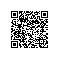# 找出运行错误的元凶

#include <iostream>
using namespace std;
class CFraction
{private:
int nume; // 分子
int deno; // 分母
public:
void input();	 //按照"nu/de"的格式，如"5/2"的形式输入
void simplify();	 //化简（使分子分母没有公因子）
void amplify(int n);	 //放大n倍，如2/3放大5倍为10/3
void output(int style);	 //输出：以8/6为例，style为0时，原样输出8/6；
//style为1时，输出化简后形式4/3；
//style为2时，输出1(1/3)形式，表示一又三分之一；
//style为3时，用小数形式输出，如1.3333；
//不给出参数和非1、2，认为是方式0
};
//主函数
int main()
{
CFraction c;
c.input();
c.simplify();
c.amplify(3);
c.output(1);
c.output(2);
c.output(3);
c.output(0);
return 0;
}
//按照"nu/de"的格式，如"5/2"的形式输入
void CFraction::input()
{
char c;
cout<<"请输入分数的值："<<endl;
while(1)
{
cin>>nume>>c>>deno;
if(c!='/')
cout<<"格式不正确，请重新输入！"<<endl;
else
break;
}
}
//化简（使分子分母没有公因子）
void CFraction::simplify()
{
int num1,num2,temp;
int x,y;
num1=nume;
num2=deno;
if(num1<num2) //找出较大的值
{
temp=num1;
num1=num2;
num2=temp;
}
x=nume;
y=deno;
while(y>0)
{
temp=x%y;
x=y;
y=temp;
};
num1=num1/x;
num2=num2/y;
cout<<num1<<'/'<<num2<<endl;
}
//放大n倍，如2/3放大5倍为10/3
void CFraction::amplify(int n)
{
int num1,num2;
num1=nume*n;
num2=deno*n;
cout<<num1<<'/'<<num2<<endl;
}
//输出：以8/6为例，style为0时，原样输出8/6；为1时，输出化简后形式4/3；为2时，输出1(1/3)形式，表示一又三分之一；为3时，用小数形式输出，如1.3333；
//不给出参数和非1、2，认为是方式0
void CFraction::output(int style)
{
if(style==1)
{
int num1,num2,temp;
int x,y;
num1=nume;
num2=deno;
if(num1<num2) //找出较大的值
{
temp=num1;
num1=num2;
num2=temp;
}
x=nume;
y=deno;
while(y>0)
{
temp=x%y;
x=y;
y=temp;
};
num1=num1/x;
num2=num2/y;
cout<<num1<<'/'<<num2<<endl;
}
else
{
if(style==2)
{
int a,b,c=0;
a=nume;
b=deno;
while(a>=b)
{
a=a-b;
c++;
}
if(a==0)
cout<<c<<endl;
else
cout<<c<<'('<<a<<'/'<<b<<')'<<endl;
}
if(style==3)
{
double c;
c=nume/deno;
cout<<c<<endl;
}
else
cout<<nume<<'/'<<deno<<endl;
}
}使用钉钉扫一扫加入圈子
+ 订阅